# Showing patterns in T-tables

### Showing patterns in T-tables

#### Lessons

In this lesson, we will learn:

• T-table are tools for keeping track of patterns and can help you predict the next terms in the pattern
• There are growing patterns and shrinking patterns involving figures and blocks/line segments/shapes or more realistic problems involving time and money

Notes:

• A T-table is a table with 2 columns (or more) that keeps track of patterns.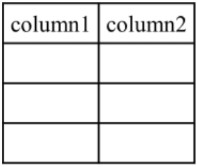• A T-table is a table with 2 columns (or more) that keeps track of patterns.
• The second column (right) keeps track of the number of things being counted (ex. blocks, line segments, dollars, etc.)

• The two types of patterns you will see in T-tables are:
• growing patterns where the numbers in the pattern are getting bigger (increasing) in each successive line of the T-table
• shrinking patterns where the numbers in the pattern are getting smaller (decreasing) in each successive line of the T-table

Example: a growing pattern with blocks starts with a 4-block figure and adds 2 each time to make the next figure has a T-table filled out like this: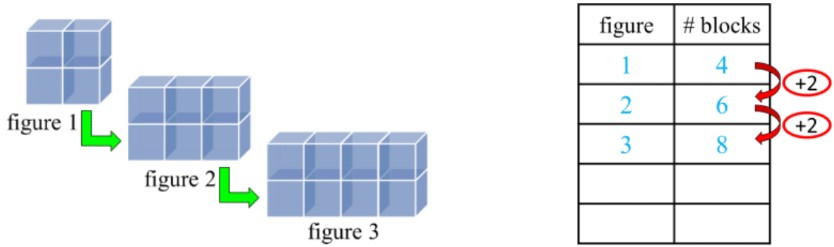• T-tables allow you to predict and calculate the next terms in the pattern
• Using the previous block example, you can continue the pattern even if you don’t have pictures of the next figures (4th and 5th figures):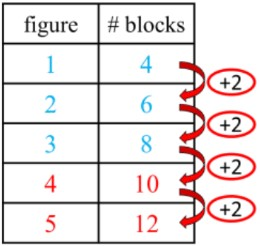• The same idea will apply for a shrinking pattern, but the relationship between each row will be subtracting an amount instead of adding.
• Introduction
Introduction to Showing Patterns in T-tables:
a)
What is a T-table?

b)
How do you show a growing pattern in a T-table?

c)
How do you show a shrinking pattern in a T-table?

d)
Summary of patterns in T-tables

• 1.
T-table block patterns: addition
The T-table shows a growing pattern of blocks. How many blocks are added to make a new figure each time?
a)
Fill in the circles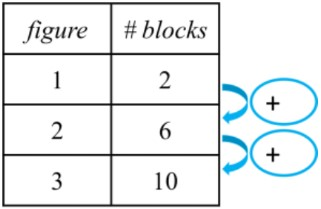b)
Fill in the circles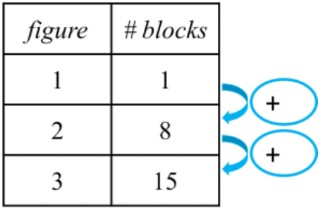• 2.
T-table block patterns: extending a growing pattern
Extend the number pattern in the T-table. How many blocks are in the 5th figure?
a)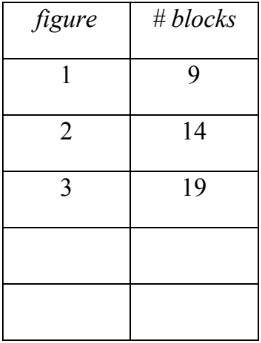b)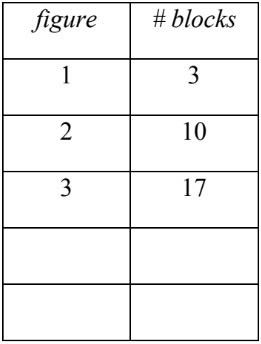• 3.
T-table line segment patterns
Complete the growing pattern by drawing line segments. Complete the next figures and fill in the rest of the T-table.
a)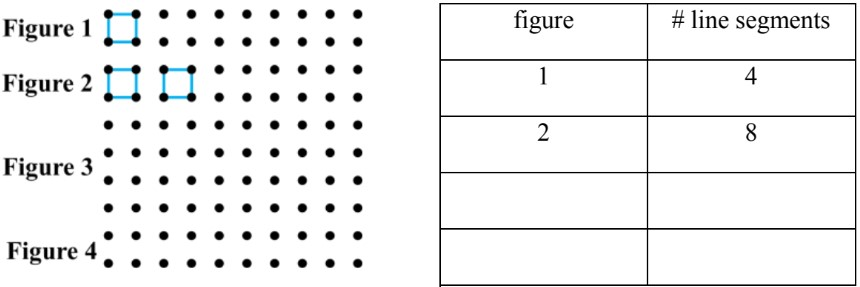b)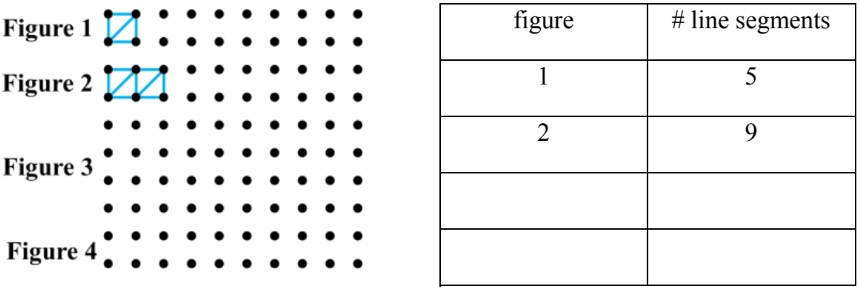• 4.
T-table patterns: word problems
Complete the T-table for the scenario and answer:
a)
If Jamie makes $11 every hour that he works at a hot dog stand, how much will he earn for 5 hours of work?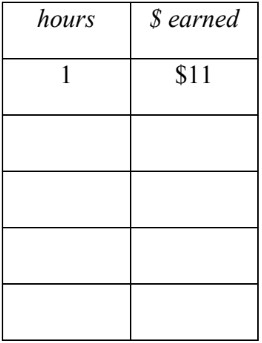b) Anna is buying trading card packs. She really wants to find a rare card! After buying her first pack, she has$37 left in her wallet. If every card pack is \$5, how much money will she have left over after buying the 5th pack?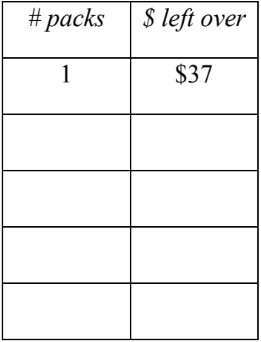c)
Garrett makes a bracelet design using trapezoids and triangles. At each stage, two triangles and a trapezoid are added. If there are 12 triangles, how many trapezoids were used?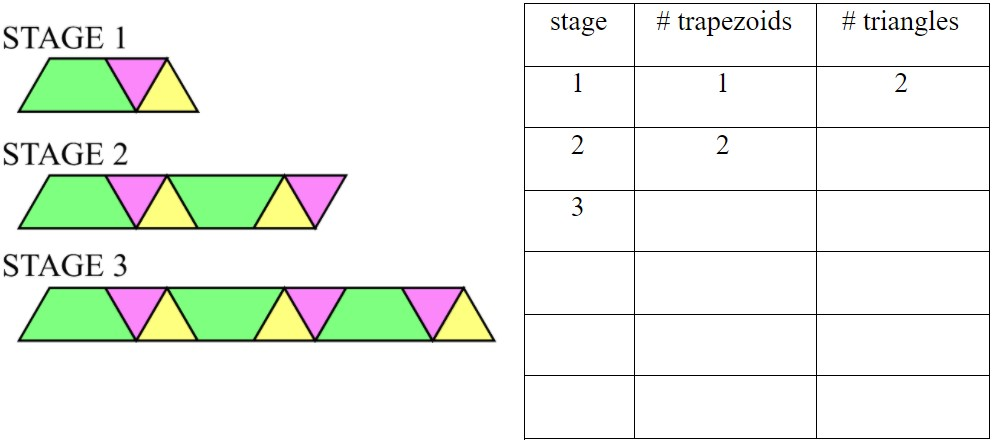d)
Kiera makes an increasing pattern with blocks. The figures in sequence use: 4 blocks, 7 blocks, 10 blocks, and then 13 blocks. After making the fourth figure, she has 15 blocks left. Does Kiera have enough blocks to make the 5th figure?